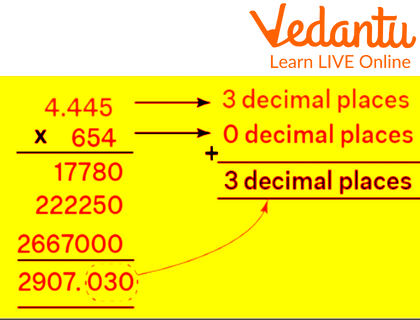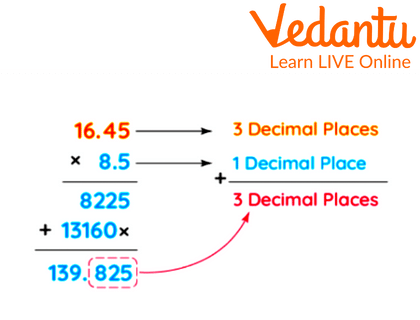Courses
Courses for Kids
Free study material
Free LIVE classes
More

# How to Multiply Decimals by Whole Numbers## Brief about How to Multiply Decimals with Whole Numbers Step by Step

Decimal multiplication is crucial when discussing the grouping of elements. Let's say there are 12 kids in all, and you have to give each one 0.25 parts of a chocolate bar. How many bars of chocolate would you require? We need to multiply 12 by 0.25 to get the required amount of chocolate bars. When multiplying decimals, one must first multiply the integers without considering the decimal point, followed by the number of decimals.

## Whole Numbers

The term "whole numbers" refers to numbers which are devoid of fractions and are made up of positive integers and zero. It is denoted by the letter "W," and its corresponding set of numbers are “0, 1, 2, 3, 4, 5, 6, 7,…”The entire number zero denotes nothing or a null value.

## Decimal Numbers

One type of number that has a whole number and a fractional component separated by a decimal point is a decimal. A decimal point is a dot that appears between parts of a whole number and a fraction. An example of a decimal number is 24.5.

## How to Multiply a Decimal with Whole Numbers Step by Step

The fundamental distinction between multiplying decimals by whole numbers and multiplying whole numbers is where the decimal point is placed. Let's examine the procedures listed below to comprehend the division of a decimal by a whole number.

Step 1: Multiply the integers using the standard approach, initially ignoring the decimal point.

Step 2: Count the number of decimal places in the given integers after the multiplication.

Step 3: The number of decimal places in the result of the multiplication should match the number provided in the numbers. In other words, the decimal point in the product should be positioned so that it equals the total of all the multiplicand and multiplier decimal places.

## Key Points to Remember

These are a few essential ideas on the idea of dividing decimals by whole numbers:

• The same guidelines apply when multiplying a decimal by a whole number as when multiplying whole integers.

• The decimal point in the product should be positioned so that it equals the total of all the multiplicand and multiplier decimal places.

• When adding the decimal point, all of the product's zeros must remain.

• Before inserting the decimal point, zeros can be added to the left if the product has more decimal places than the number of digits.

• The final product's trailing zeros can be removed.

## Conclusion

One of the crucial applications is multiplying a decimal by a whole number when billing for things where the amount is frequently expressed in decimals. We need decimals in our daily life. The majorities of their applications are in industries like measurement and finance where precise computations are required.

## Solved Examples

Example 1: Salina is attempting to calculate the product of two numbers, 654 and 4.445. Can you help her in doing the multiplication?

Ans: We can see that the numbers are 654 and 4.445, which are decimals and whole numbers, respectively.

We will thus use the procedures for multiplying a decimal by a whole number in order to obtain the product of these two values.Multiplication of 4.445 and 654

Therefore, 4.445 multiplied by 654 results in 2907.03

Example 2: If Raj got 88.63 marks in English, Math and Hindi. Then how many marks did Raj get in these three subjects? (Use multiplication)

Ans: Given that Raj got 88.63 marks in 3 subjects.

Thus, his marks in total in these 3 subjects would be $88.63 \times 3 = 265.89$

Total marks he got is 265.89.

Example 3: Find the product of 16.45 and 8.5.

Ans:Multiplication of 16.45 and 8.5

Last updated date: 05th Jun 2023
Total views: 47.4k
Views today: 1.42k

## FAQs on How to Multiply Decimals by Whole Numbers

1. How do you divide a decimal by a 1000?

Since there are three zeros in 1000, you must move the decimal point three spaces to the right in order to multiply decimals by 1000.

2. Does multiplication with decimals require them to be lined up?

Arrange the numbers vertically so that each number's rightmost digits are aligned. Just align the right-most digits; do not align the decimal points.

3. How will we use decimal multiplication in practical situations?

Every day, while dealing with things like money, weight, and length, we employ decimals.

4. What happens if we multiply any number by 0?

If 0 is multiplied by any number, the result will always be 0.